# Treefit

## The first software for quantitative trajectory inference

Treefit is a novel data analysis toolkit that helps you perform two types of quantitative analysis: 1) measuring the goodness-of-fit between your single-cell RNA-seq data and esimated tree trajectories; and 2) discovering novel cell types or detecting contaminating cell types by predicting the number of principal paths in the best-fit tree trajectory. Treefit can be used to analyze either row counts or normalized expression data.

Treefit is implemented in both R and Python languages and can be used in conjunction with other popular software packages, such as Seurat and dynverse. There are user-friendly tutorials of Treefit.

### 1) Measuring the goodness-of-fit between data and data-derived tree trajectories

Trajectory inference is one of the key computational challenges in single-cell transcriptomics. In the last few years, many software packages have been developed and widely used to extract an underlying "tree" structure from single-cell RNA-seq data.

However, trajectory inference often suffers from the uncertainty due to the heterogeneity of individual cells and the high levels of technical noise in single-cell experiments. Therefore, in order to facilitate scientific discoveries, it is crucial to establish a theoretically sound quantitative method that is underpinned by a mathematical/statistical evidence base.

Treefit is a library to examine the reliability of inferred trajectories based on the robustness of the tree structures.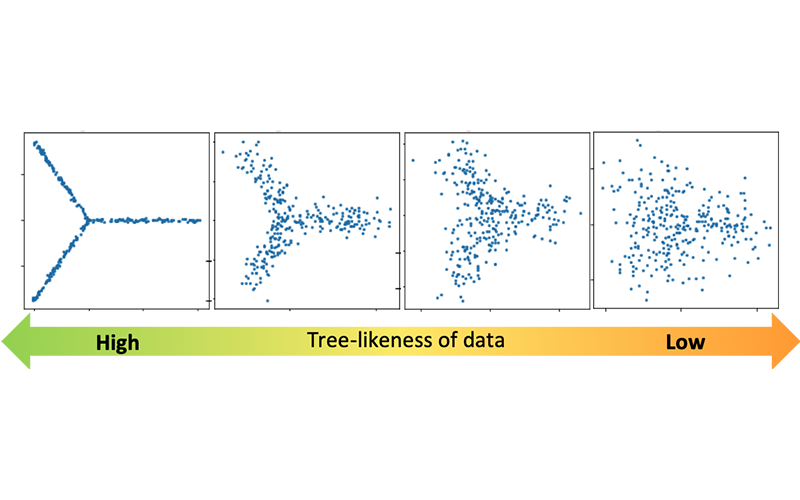### 2) Estimating the number of "principal paths" in the best-fit tree trajectory

Single-cell technologies are expected to help us discover a novel type of cells and revolutionize our understanding of the process of cell differentiation; however, it is not always easy to distinguish distinct types of cells or to quantify how different their gene expression profiles are.

Many past studies involved exploratory data analysis relying on visualization. However, using different visualization techniques can produce completely different pictures and the interpretation can be subjective. Therefore, there has been an urgent need for a more objective, reliable methodology in order to directly reach a scientific truth.

With help of powerful techniques in various disciplines of mathematics, Treefit gives insight into the "shape" of your data (e.g. linear maturation, bifurcation, or more complex divergence) and helps accelerate scientific discoveries in a mathematically and statistically valid way.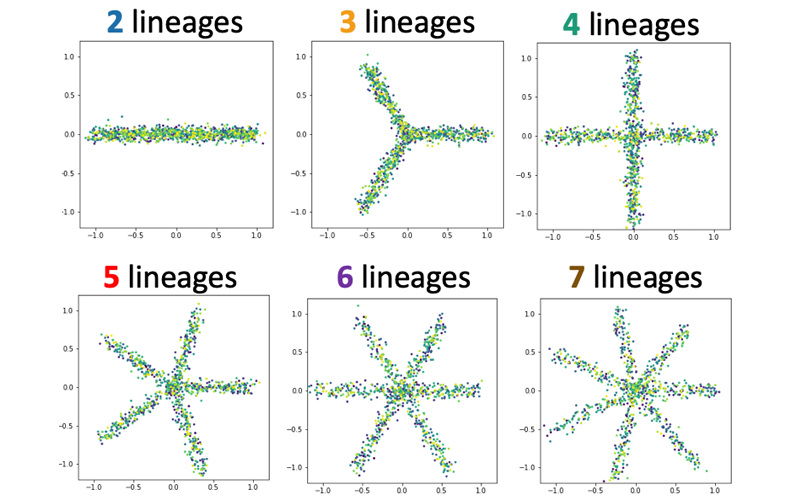## Theoretical ideas

One of the fundamental beliefs in cell biology is that the process of cell differentiation can be modeled by a "tree" (i.e., connected graph without a cycle). However, it depends on the situation whether or not this assumption is valid. Indeed, some data are too noisy to be exactly depicted by a tree and some cells are known to undergo dedifferentiation that is not a tree-like but cycle-like process. Treefit verifies how well your data can be explained by a tree model and thus helps you perform quantitative trajectory inference.

### 1. Adding small noises to original data

In mathematics, perturbation refers to the operation of adding a slight shift to a number. In our context, it means adding small noises to the values of original gene expression data. If you input a gene expression data matrix, then Treefit repeatedly perturbs the data so that you can obtain many possible datasets without actually conducting the same single-cell experiments over and over again.

Treefit currently supports two types of perturbation, one of which uses the statistical uncertainty of the raw counts while the other employs an interpolating techniques that can also be applied to processed data.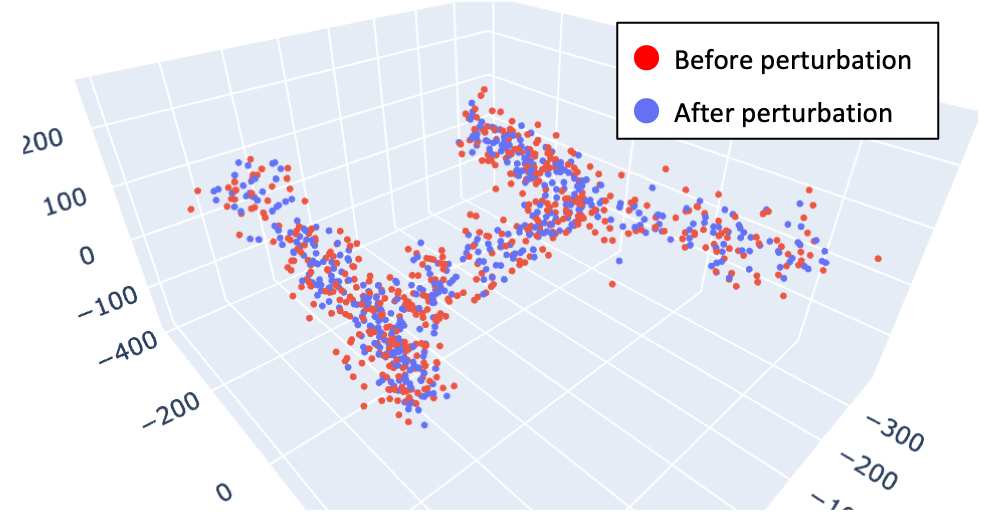### 2. Measuring the robustness of inferred tree trajectories

Once creating many datasets by simulating different kinds of noise, we can build many possible trees by applying any tree trajectory inference method to each gene expression data matrix. Treefit calculates a tree for each realized expression matrix, then compares their global structures using geometrical methods. The current version of Treefit computes a minimum spanning tree (MST) because many existing trajectory inference software packages uses MSTs.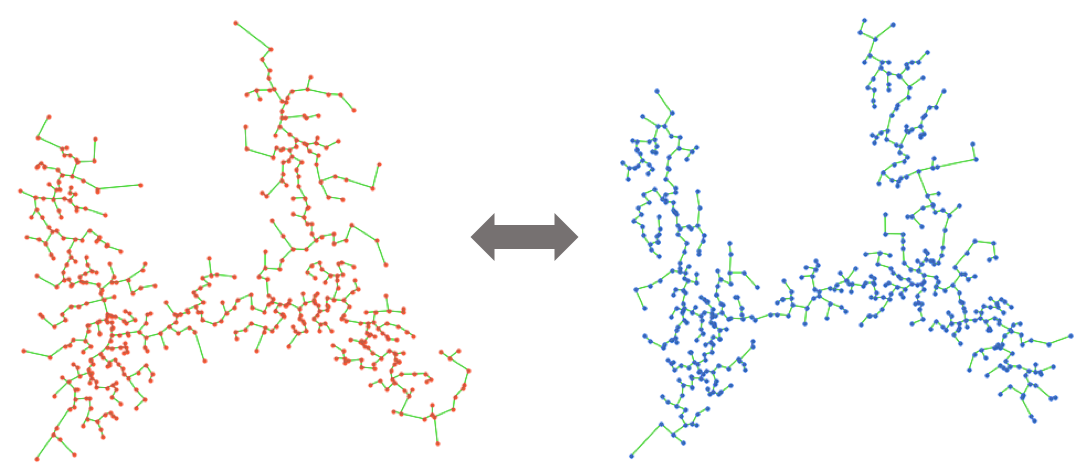Treefit analyzes the goodness-of-fit between data and data-derived tree models by measuring the structural similarity between many inferred tree trajectories. In principle, the tree structure is stable when data have an intrinsic tree-like topology, while it becomes unstable for non-tree-like data (e.g., cycle, diffusive).The analysis of two artificial datasets shows that the structure of MSTs is robust to noise when the shape of data is tree-like but is not in the cycle-like case (note that longest paths are highlighted for illustrative purposes here).

### 3. Grassmann manifolds of Laplacian eigenseries

As the measure of the similarity of global structures between trees, Treefit calculates the eigenseries of the graph Laplacian for each tree, and then computes the distance between the spaces spanned by the leading eigenvectors, which represents the low-frequency components of the graph topology.

Treefit supports several distance measures with different definitions in order to help extract diverse information about the topology of the data.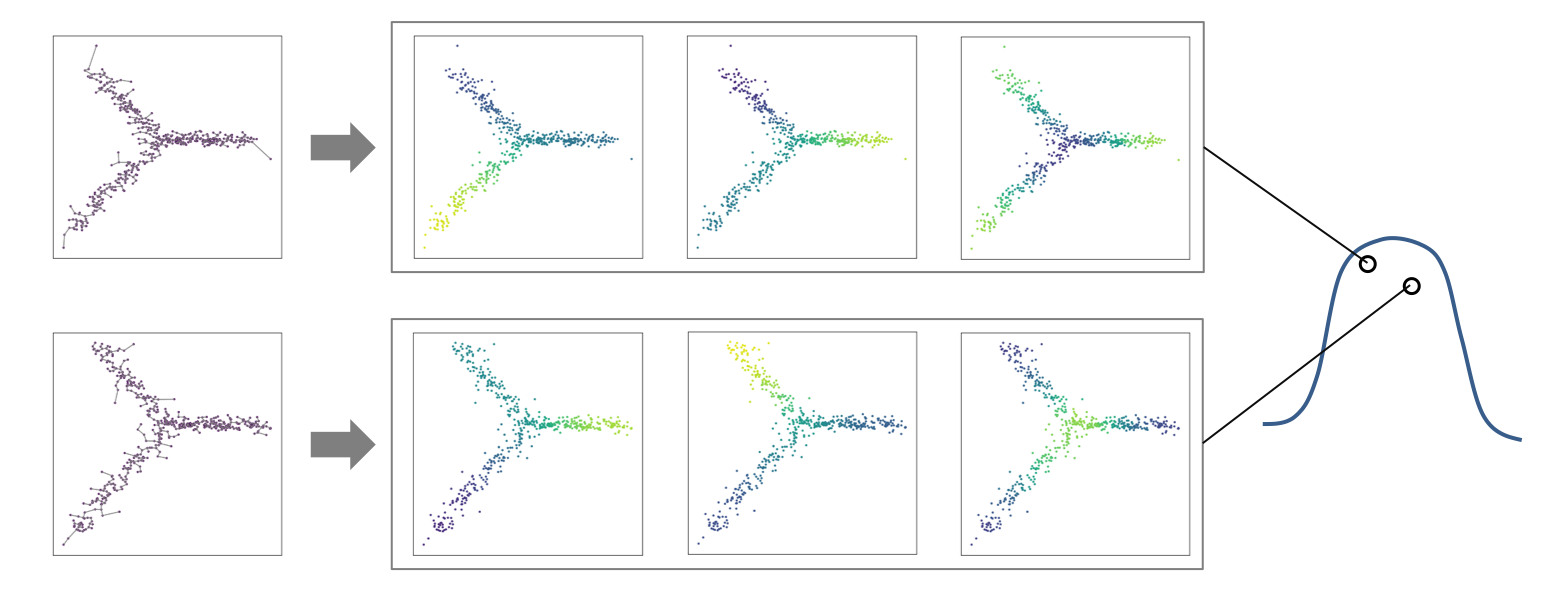## Getting started

There are Treefit for R and Treefit for Python.